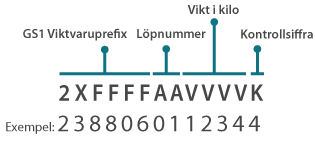How do I create my variable weight numbers?

The variable weight number is a 13-digit number that you create using the GS1 Variable Weight prefix, a serial number, the price in Swedish crowns (sek) or the weight in kilograms plus a check digit. Then use the data carrier EAN-13 to label your products with variable weight.

GS1 Weight product prefix consists of 5-6 digits, depending on how many items your company has in the range. The weight item prefix always starts with 2X, and you set X to 0, 1, 2, 3, 4 or 5 depending on whether you notice with weight or price:

0 = Price in sek with two decimals (e.g. 1.20 kr)

1 = Price in sek with a decimals (e.g. 12.40 kr)

2 = Price in sek, integer (e.g. 1234 kr)

3 = Weight in kilograms with three decimals (e.g 1.234 kg)

4 = Weight in kilograms with two decimals (e.g. 12.34 kg)

5 = Weight in kilograms with one decimals (e.g. 123.4 kg)

After the weight ing prefix follows a serial number consisting of 2-3 digits. It allows you to number from 100 up to 1,000 items. For a weight-item prefix with 6 digits, running numbering means that the first item is numbered “… 01”, the second article “… 02” and so on.

After the serial number, the price is in crowns or the weight in kilograms.

Last comes the check digit, which you calculate using our calculator.